MATHGOTSERVEDPRECALCULUS
UNIT 5: TRIGONOMETRIC IDENTITIES
ANALYTIC TRIG
5-1 Fundamental Trig Identities
The Fundamental Trig Identities
The fundamental trig identities are the reciprocal, quotient, pythagorean, co-function and odd/even identities.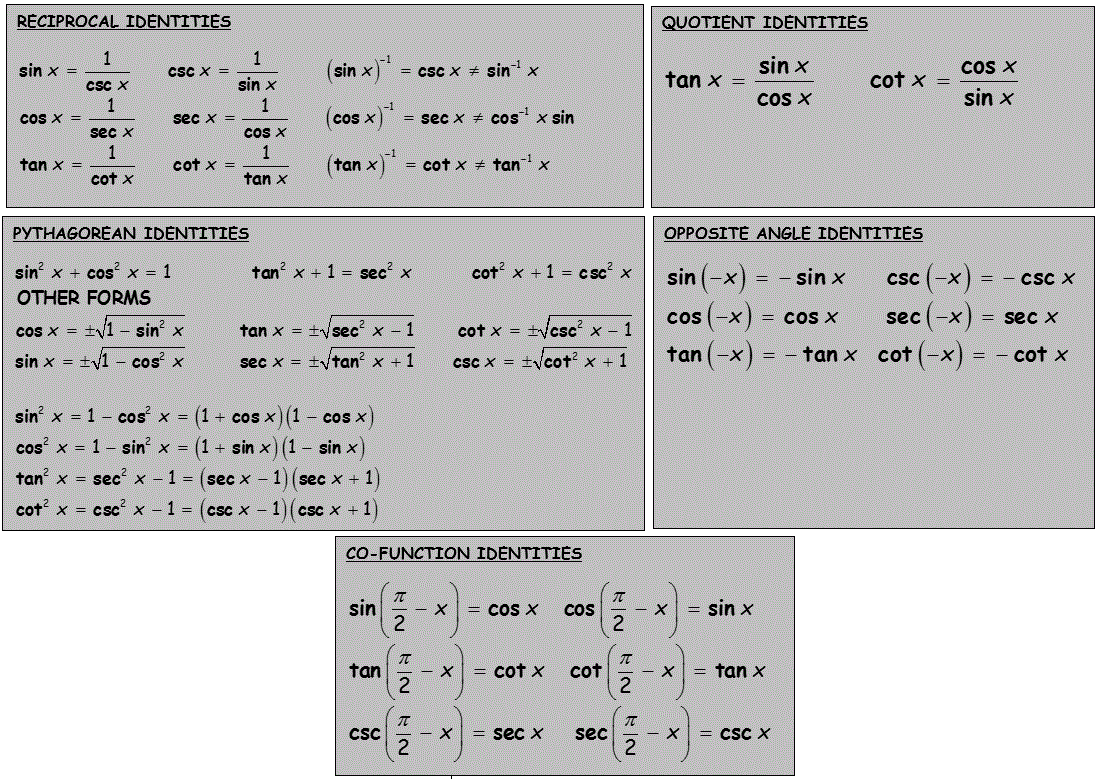Examples on Fundamental Trig Identities
1.2.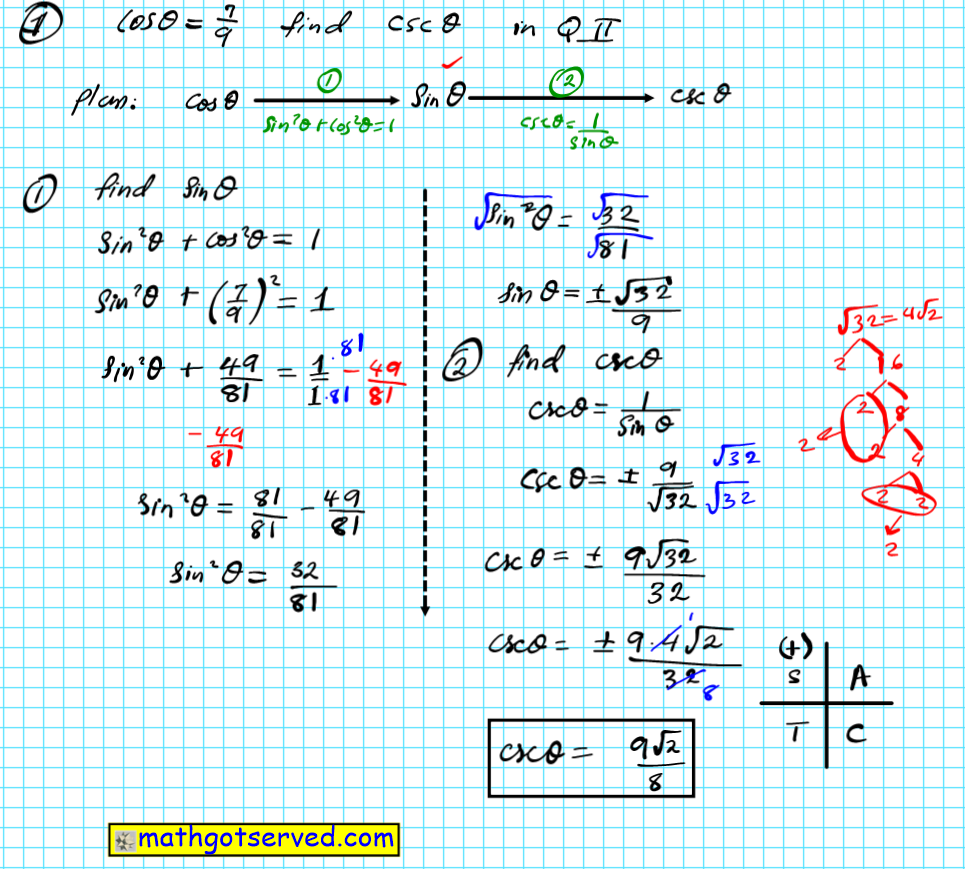3.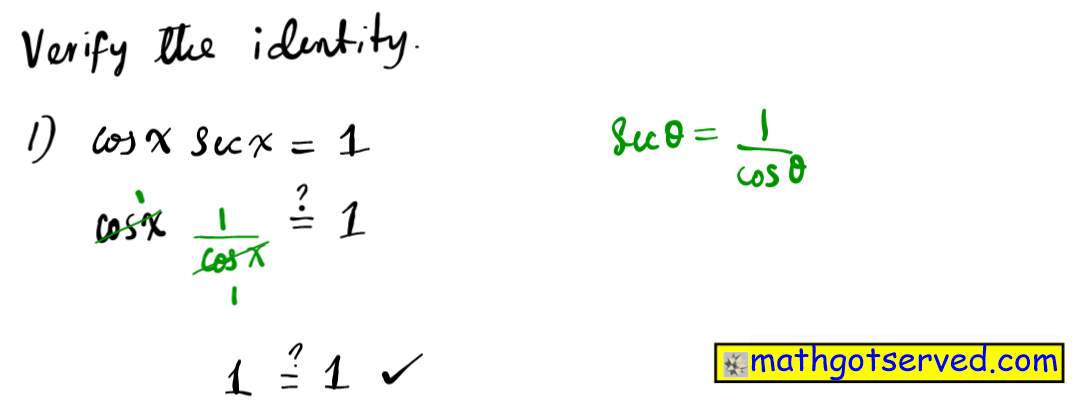4.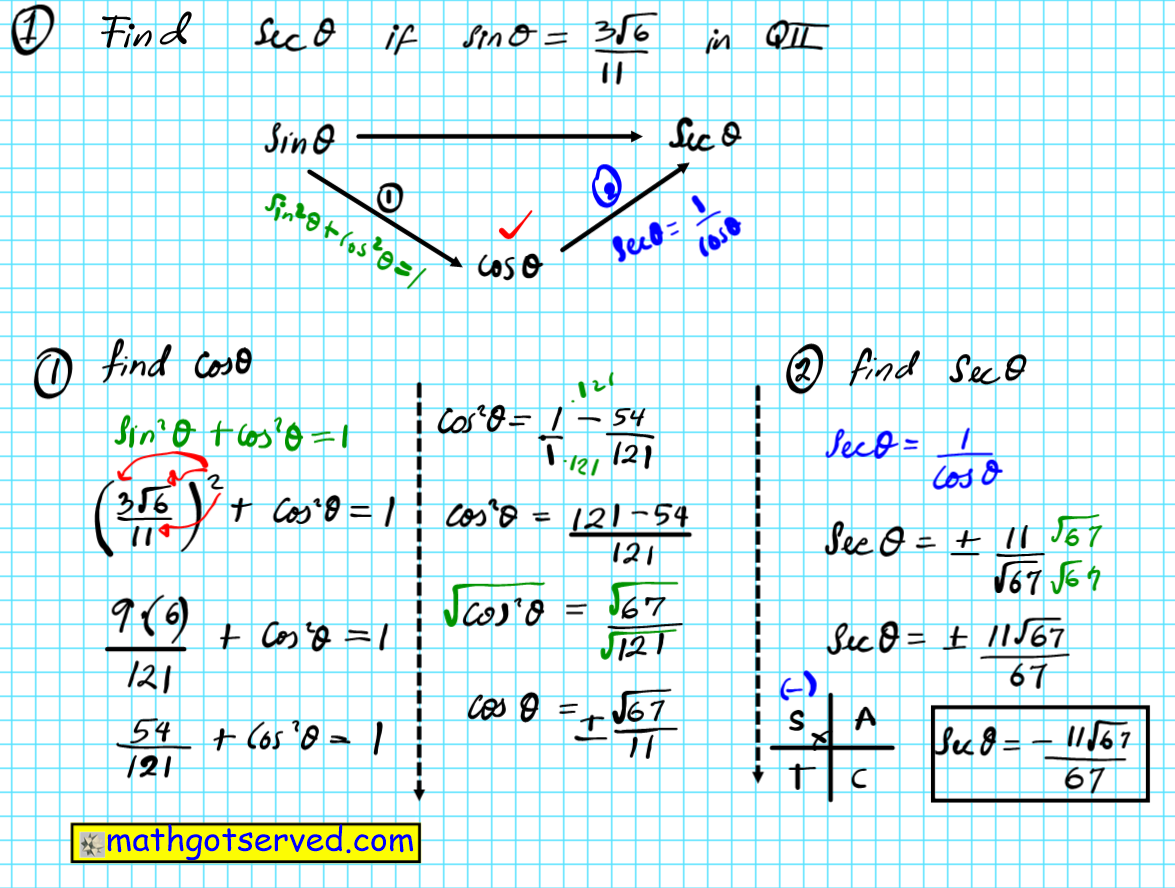5.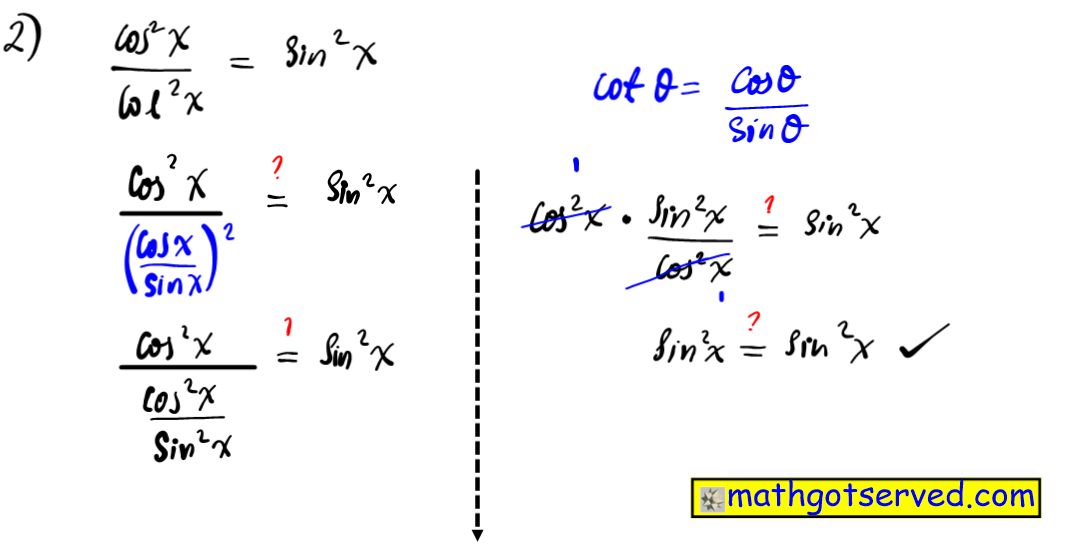6.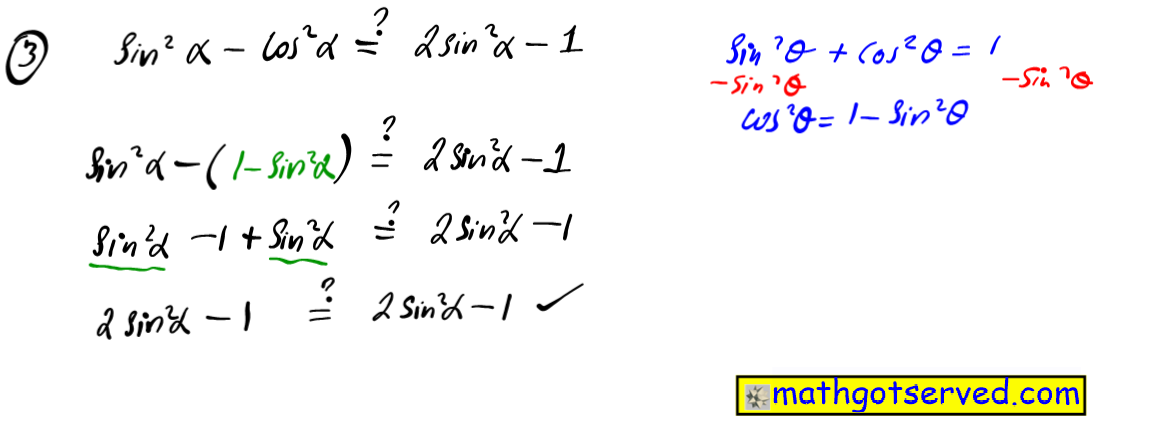7.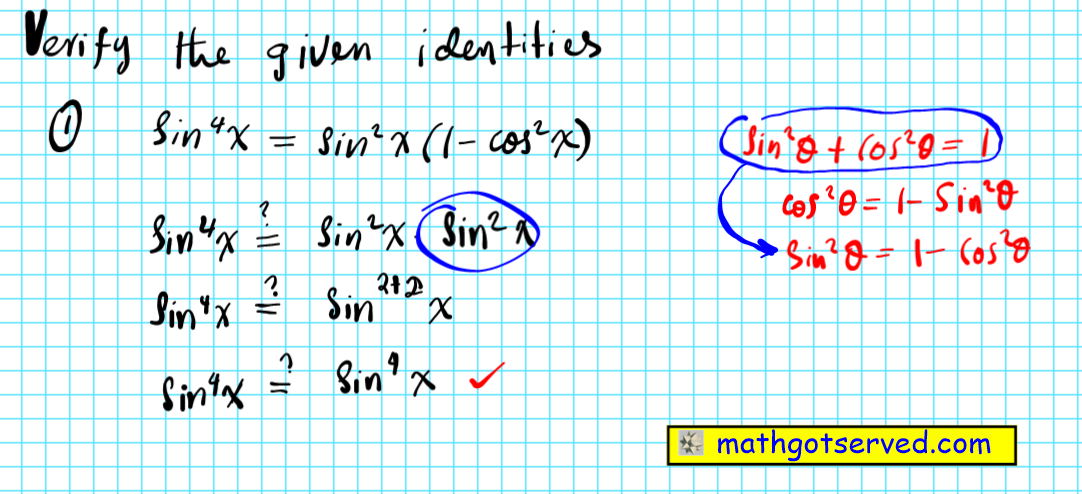8.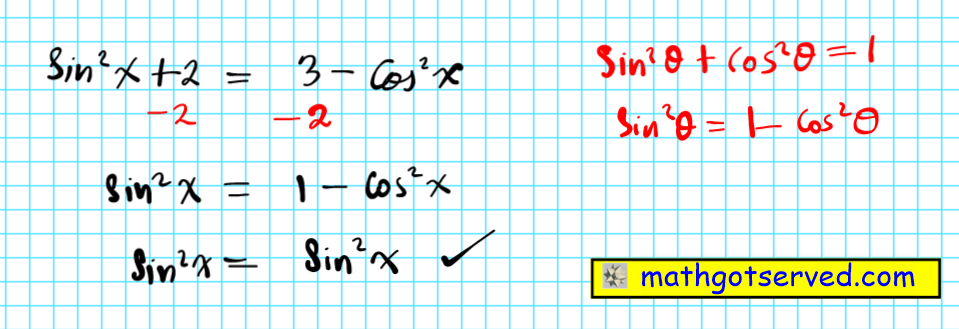9.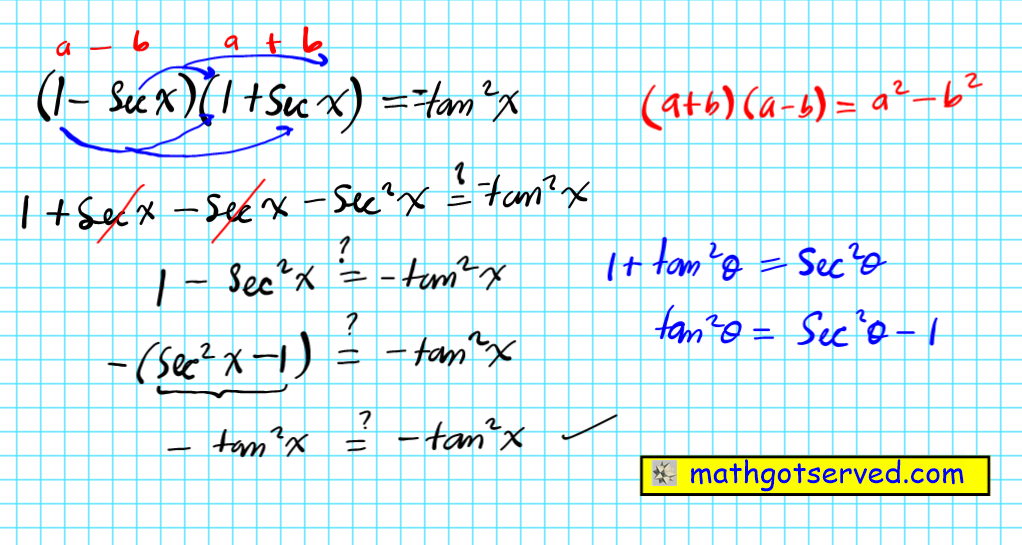10.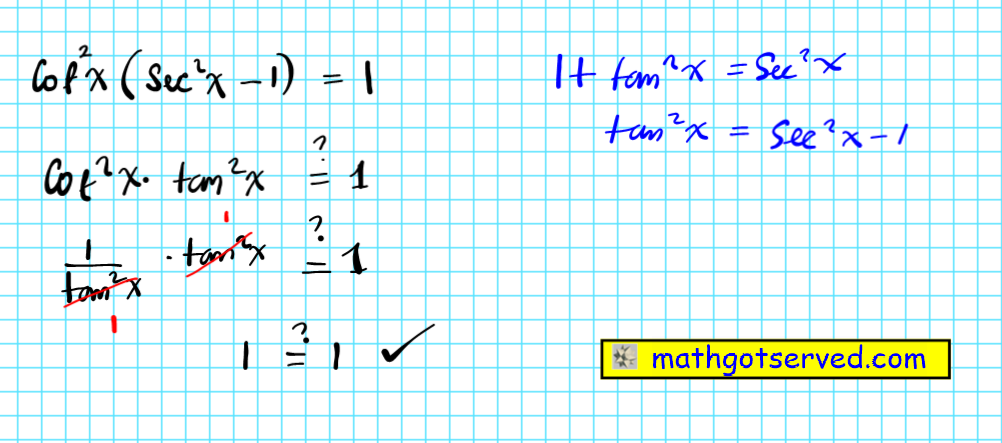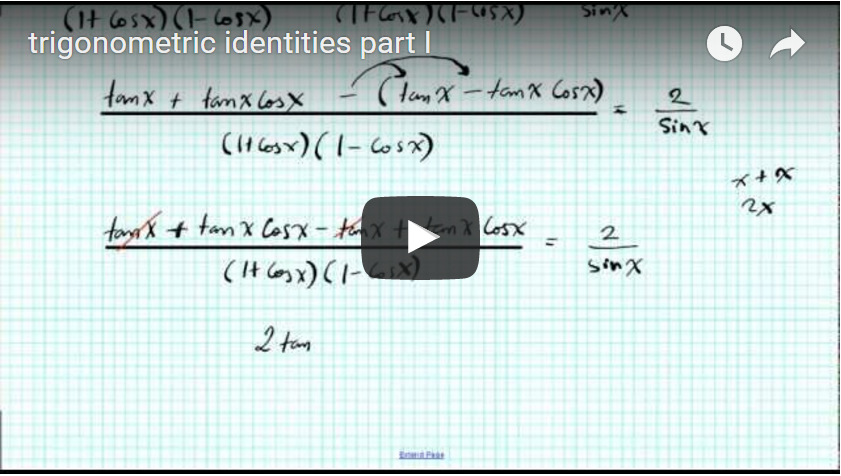5-3 Solving Trig Equations
5-4 SUM AND DIFFERENCE IDENTITIES
Some angles that are not on the unit circle can be expressed as the sum or difference of angles on the unit circle. Applying the sum and difference identities to these triangles makes it possible to find the exact value of these triangles for all six trig functions.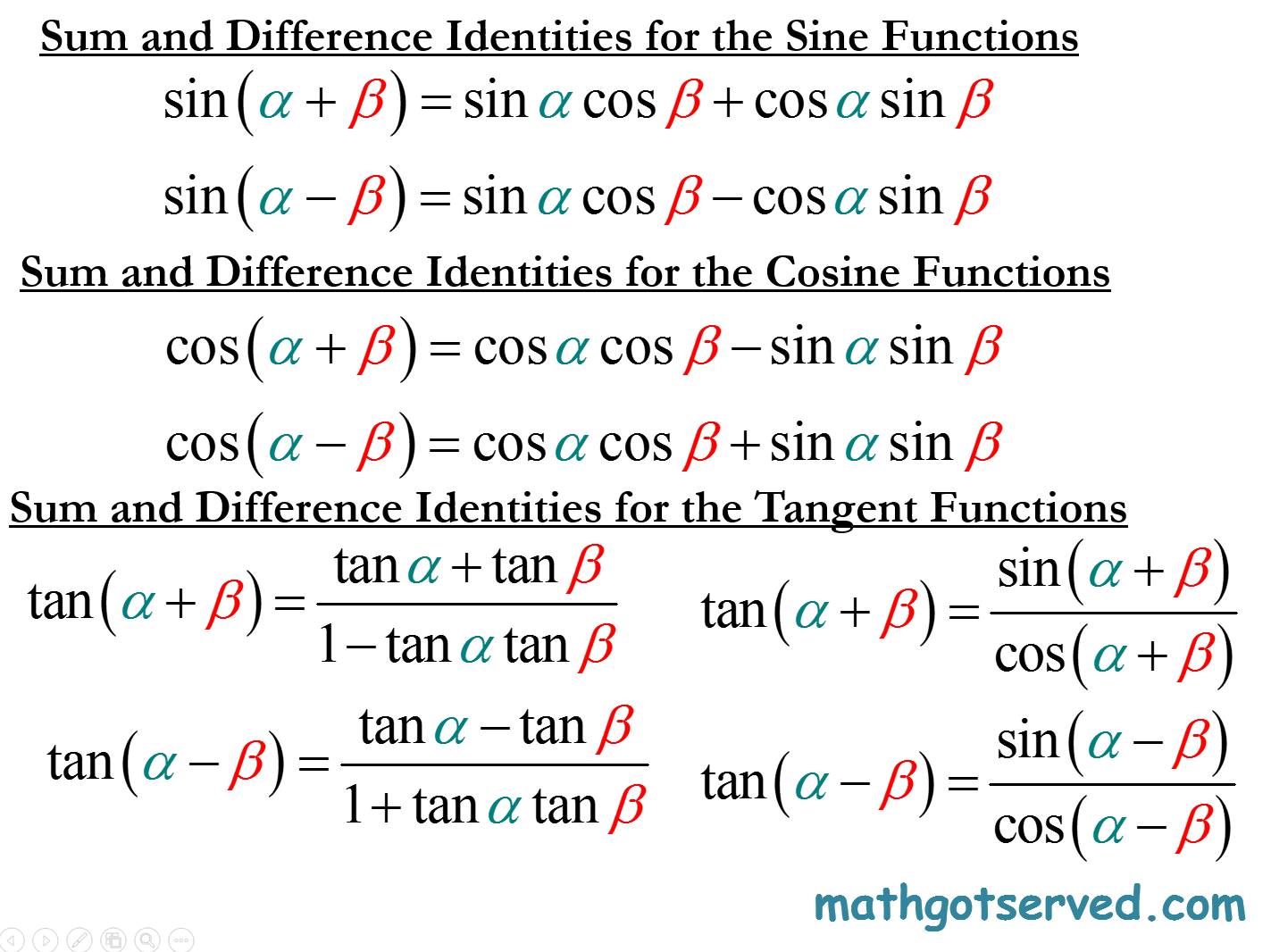5-5 MULTIPLE ANGLE IDENTITIES (HALF AND DOUBLE ANGLE IDENTITIES